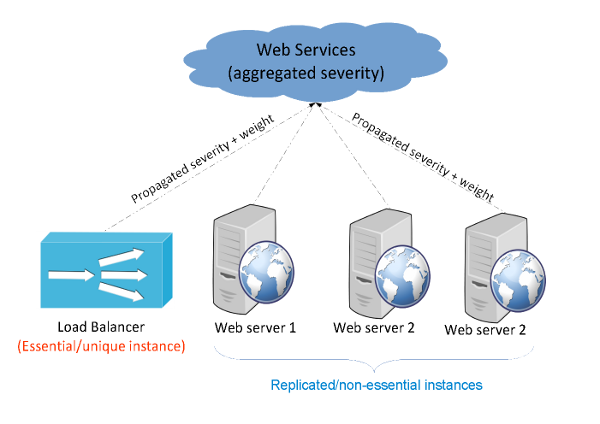# Reference - Dive into Severity Calculation and Propagation

severity-calculation_and_propagation

This page provides deep details on how RealOpInsight handle severities on services it manages. The severity management is underlyng by two basic concepts: severity calculation on each node and severity propagation to parent service.

## What are Severity Calculation and Propagation?

Let consider a business service dependency tree corresponding to a website architecture depicted on the figure hereafter. In the architecture, we consider a load balancer and three web servers working as replicated request handlers behind the load balancer. So, let `My Website` be the business service representing the health of the website, `Load Balancer` be the load balancer business service, `Web Server1`, `Web Server2` and `Web Server3` be respectively the business services representing the different web servers. Basing on the associated business service tree, we’ll present below four use cases to illustrate how status aggregation works.## Example 1: All Essential Services in Normal State

Consider that:

• the load balancer is an essential service and is in the Normal state;
• the other services (Web Server1, Web Server2 and Web Server3) are all non-essential and have the same weight w=1, while being respectively in the states Normal, Minor and Major;
• the calculation rule used to compute the status of the website is Weighted Average

Hence, the maximum severity of essential services is Normal while the weighted average of severities propagated by non-essential shall be:

``````nonessential_weighted_average_severity = ROUND (1*0 + 1*1 + 1 * 2) / (1 + 1 + 1) = 1
``````

Finally, the overall severity of the `My Website` shall be equal to `MAX (Normal, Minor) = Minor`.

## Example 2: Essential Services in Non-Normal State

From the previous example, now consider that the load balancer is in Critical state and that the other services have the same statuses as previously (i.e. respectively Normal, Minor, Major).

Hence, the overall severity of the ``My Website` service shall be equal to `MAX (Critical, Minor) = Critical`

## Example 3: Thresholds and Status Escalation

Now consider that:

• the load balancer is still working as essential service and has the severity Normal;
• the other services (Web Server1, Web Server2 and Web Server3) are all non-essential and have the same weight w=1, while being respectively in the states Normal, Minor and Minor;
• the calculation rule used to compute the overall severity of the `My Website` service is Weighed Average With Thresholds, knowing that the following threshold rules have been defined: `50% of Minor => Major`, `60% of Major => Critical`.

Hence:

• the maximum of severity of essential services shall be Normal;

• the weighed average of severities of non-essential subservices shall be:

``````nonessential_weighted_average_severity = ROUND ( (1*0 + 1*1 + 1*1) / (1 + 1 +1 ) = 1 = Minor
``````
• the percentage of non-essential services in Minor state is 2/3 ~= 67%, meaning that the first threshold is exceeded. Therefore the status shall be escalated from Minor to Major.

• The overall severity of the `My Website` service shall be equal `MAX (Normal, Minor, Major) = Major`, due to the exceeded threshold.

## Example 4: Thresholds, Weighting and Status Escalation

We consider in this use case that:

• the load balancer is an essential service and is in Normal state;
• the services ``Web Server1` and `Web Server2` have the same weight w1=w2=1 and are all in Normal state;
• the service `Web Server2` has the weight w3=4 and is in Major state.
• the calculation rule used to compute the overall severity of the `My Website` service is Weighed Average With Thresholds, knowing that the following threshold rules have been set: `50% of Minor => Major`, `60% of Major => Critical`.

Hence:

• the maximum of severity of essential services shall be Normal;

• the weighed average of severities of non-essential subservices shall be:

``````nonessential_weighted_average_severity = ROUND ( (1*0 + 1*0 + 2*4) / (1 + 1 + 4 ) = 1 = Minor
``````
• the weighted percentage of non-essential services in Major state is 4/6 ~= 67%, meaning that the second threshold is exceeded. Hence the status shall be escalated from Major to Critical.

• The overall severity of the `My Website` service shall be equal to `MAX (Normal, Minor, Critical) = Critical`, due to the exceeded threshold.